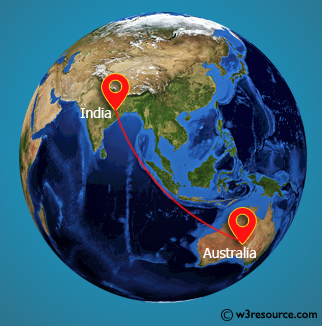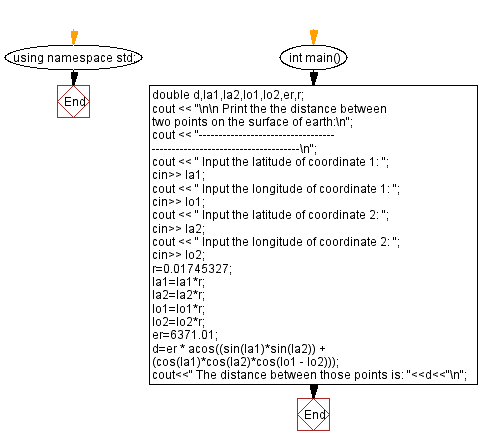﻿ C++ : Distance between two points on the surface of earth

# C++ Exercises: Compute the distance between two points on the surface of earth

## C++ Basic: Exercise-59 with Solution

Write a C++ program to compute the distance between two points on the surface of the earth.

Pictorial Presentation:Sample Solution:

C++ Code :

``````#include <iostream>
#include <math.h>
using namespace std;

int main()
{
double d,la1,la2,lo1,lo2,er,r;
cout << "\n\n Print the the distance between two points on the surface of earth:\n";
cout << "-----------------------------------------------------------------------\n";
cout << " Input the latitude of coordinate 1: ";
cin>> la1;
cout << " Input the longitude of coordinate 1: ";
cin>> lo1;
cout << " Input the latitude of coordinate 2: ";
cin>> la2;
cout << " Input the longitude of coordinate 2: ";
cin>> lo2;
r=0.01745327; //Pi/180=3.14159/180
la1=la1*r;
la2=la2*r;
lo1=lo1*r;
lo2=lo2*r;
er=6371.01; //Kilometers
d=er * acos((sin(la1)*sin(la2)) + (cos(la1)*cos(la2)*cos(lo1 - lo2)));
cout<<" The distance between those points is: "<<d<<"\n";
}
``````

Sample Output:

``` Print the the distance between two points on the surface of earth:
-----------------------------------------------------------------------
Input the latitude of coordinate 1: 25
Input the longitude of coordinate 1: 35
Input the latitude of coordinate 2: 35.5
Input the longitude of coordinate 2: 25.5
The distance between those points is: 1480.08
```

Flowchart:C++ Code Editor:

What is the difficulty level of this exercise?

﻿

## C++ Programming: Tips of the Day

Why is there no std::stou?

The most pat answer would be that the C library has no corresponding "strtou", and the C++11 string functions are all just thinly veiled wrappers around the C library functions: The std::sto* functions mirror strto*, and the std::to_string functions use sprintf.

Ref: https://bit.ly/3wtz2qA

We are closing our Disqus commenting system for some maintenanace issues. You may write to us at reach[at]yahoo[dot]com or visit us at Facebook